# Gromov hyperbolic space

Jump to: navigation, search

hyperbolic space in the sense of Gromov

This notion provides a uniform "global" approach to such objects as the hyperbolic plane, simply-connected Riemannian manifolds with pinched negative sectional curvature,-spaces, and metric trees. Various "hyperbolic properties" introduced earlier (mostly in the context of group theory) [a1], [a2], [a3] were summed up and further developed by M. Gromov in his seminal paper [a4]. More detailed expositions of (some parts of) Gromov's work can be found in [a5] and [a6].

Ifis a metric space, then the Gromov product of two points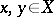with respect to a reference point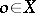is defined as(whenis a tree, this product coincides with the confluent ofand, i.e., the length of the common part of the geodesic segments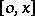and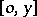). The spaceis called-hyperbolic (with a constant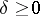) if the Gromov product satisfies the-ultrametric inequalityfor anypoints. Ifis-hyperbolic for some, it is called Gromov hyperbolic. Any metric tree is-hyperbolic, and, conversely, any-hyperbolic space isometrically imbeds into a tree. For Cartan–Hadamard manifolds, hyperbolicity is equivalent to the strong visibility property [a7].

Often one adds to the definition of Gromov hyperbolicity the following two requirements: 1)is geodesic (cf. also Geodesic manifold), i.e., any two points incan be joined by a path (a geodesic) whose length coincides with the distance between these points; and 2)is proper, i.e., all metric balls inare compact. Then hyperbolicity is equivalent to the following thin triangles condition: there exists a constant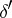such that for any geodesic triangle inone can choose a point on each of the sides of the triangle in such a way that the pairwise distances between thesepoints are all not greater than.

Yet another definition of hyperbolicity can be given in terms of exponential divergence of geodesic rays in, cf. [a8].

A sequence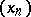inis called convergent if. The hyperbolic boundary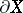of a hyperbolic spaceis defined as the set of equivalence classes of convergent sequences with respect to the equivalence relation. Any geodesic ray inis convergent, and, conversely, for any class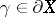and any pointthere exists a geodesic ray (not necessarily unique!) joiningand, i.e., starting fromand belonging to the class. Equivalently,can be defined as the set of asymptotic classes of geodesic rays, with two rays being asymptotic if they are within bounded distance from each other.

The hyperbolic boundary is the boundary of the hyperbolic compactification of. For trees the hyperbolic compactification coincides with the end compactification, and for hyperbolic Cartan–Hadamard manifolds with the visibility compactification. The Gromov product naturally extends to, and, for sufficiently small, is uniformly equivalent to a metric on.

Hyperbolicity of a metric spaceis determined by its "global" structure only. If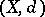andare two metric spaces such that there exist mappingsand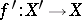and a constant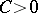with the property that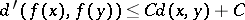and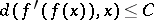for all, andandfor all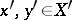(such metric spaces are called roughly isometric), thenand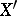are hyperbolic simultaneously, and the hyperbolic boundariesandare homeomorphic.

Isometries of hyperbolic spaces can be classified into elliptic, parabolic and hyperbolic ones in the same way as isometries of the usual hyperbolic plane. An important class of hyperbolic spaces is provided by finitely generated groups whose Cayley graphs are Gromov hyperbolic spaces (such groups are called word hyperbolic).

See also Hyperbolic group.